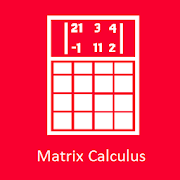# Matrix CalculusEveryone
2
Offers in-app purchases
Matrix Calculus is the best current application for mathematical operations involving numbers, matrices and multi-dimensional matrices;
it’s able to perform all standard mathematical calculations on numbers, vectors (matrices of size 1) and matrices from 2 to 5 dimensions.
The only limits are the following:
- Dimensions of a matrix from 1 to 5
- Maximum total length of a matrix less than 3200
- Maximum length of a matrix dimension = 50

The possible operations are the standard of mathematics and the following matrix operations:

* = product matrix
/ = division of two matrices, or product of the inverse matrix
^ = power of a matrix (only with integers)
+ = sum matrix
- = difference matrix
Det = Determinant
Tra = matrix transpose
Inv = matrix inverse
Adj = adjoint matrix
tr(A) = trace of matrix A
Unit = matrix unit
Rank = matrix rank
Erf = error function erf
REF = matrix in Row Echelon Form (system solution)
The following matrix operations are operative only with the Pro version:
Inv+ = Moore - Penrose pseudo inverse
Eigen = matrix eigenvalues
Evect = matrix eigenvectors
Vsing = matrix singular values S
Uvect = left vector singular matrix U
Vvect = right vector singular matrix V
Dsum = matrix direct sum
Outer = outer product
L(L*L’) = Lower triangolar matrix L so that A = L*L’
Q(Q*R) = Left matrix Q so that A = Q*R
R(Q*R) = Wright matrix R so thar A = Q*R
Jordan = Jordan matrix J
||A|| = Frobenius norm
e^A = exponential of matrix A
√ A = square root matrix

If the matrix allows, it is also possible to calculate a matrix function, where the function is one of those of the calculator, for example (A = matrix):
lne (A), log (A), sin (A) cos (A), tan (A), sinh (A), arcsin (A), arctanh (A)
Read more
Collapse

Review Policy
5.0
2 total
5
4
3
2
1
Loading...

## What's New

Added 64bit version
Read more
Collapse

## Additional Information

Updated
May 27, 2019
Size
34M
Installs
50+
Current Version
5.15
Requires Android
6.0 and up
Content Rating
Everyone
In-app Products
\$0.99 - \$1.49 per item
Permissions
Offered By
IngSM
Developer
Frazione Moron Hugonet, 12 11027 Saint-Vincent Italy
©2019 GoogleSite Terms of ServicePrivacyDevelopersArtistsAbout Google|Location: United StatesLanguage: English (United States)
By purchasing this item, you are transacting with Google Payments and agreeing to the Google Payments Terms of Service and Privacy Notice.